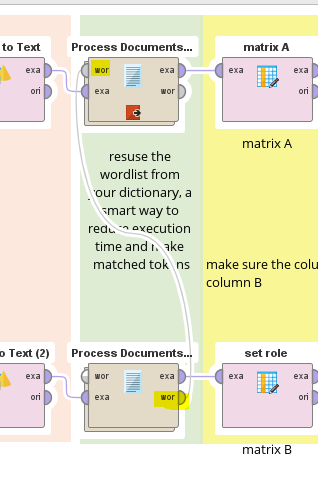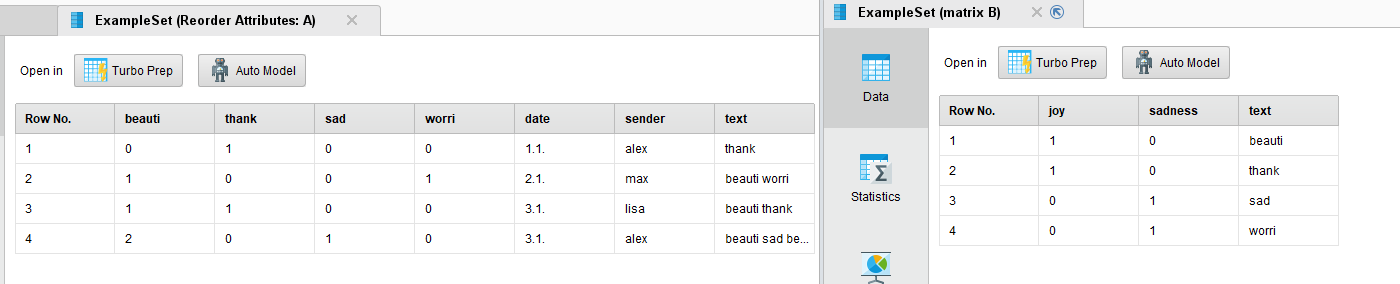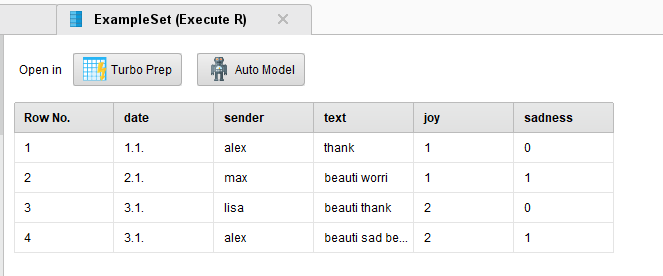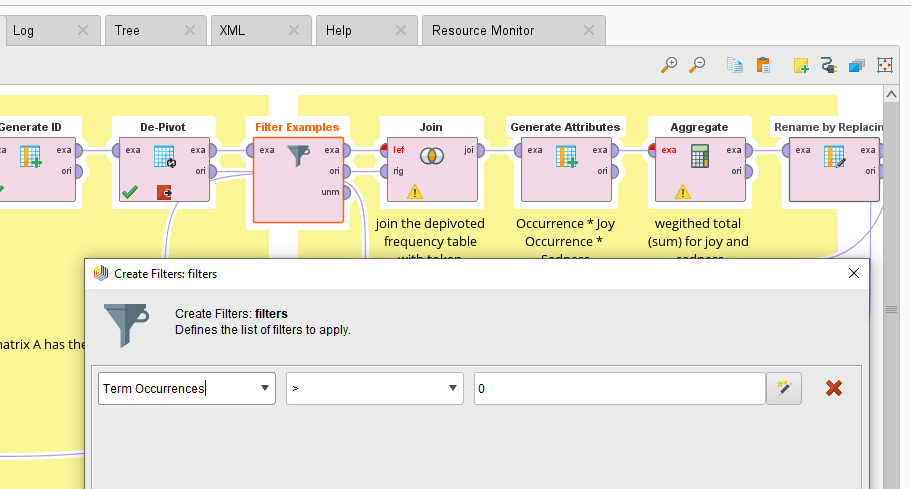# comparing two datasets and calculating multiple values

Member Posts: 3Learner I
Hello there,

I'm doing some kind of dictionary-based emotion-analysis in which I want to compare a set of text messages against a dictionary. The text message dataset consist of the three attributes "date", "sender" and the "message" itself. The dictionary marks the corresponding emotion for each word with a boolean marker like the following:

 word emo1 emo2 emo3 w1 1 0 1 w2 1 0 0 w3 0 1 0 w4 0 1 1

After processing the text messages and the lexicon (cases, stopwords, tokenize, stem), I end up with a word vector for the text message dataset that displays the count of each word for every message:

 date sender w1 w2 w3 w4 1.1. alex 0 0 1 0 2.1. max 1 0 0 1 3.1. lisa 1 0 1 0 3.1. alex 2 1 0 0

My goal is to create a table in which a score for each of the emotions is calculated for every message. To do so, the corresponding words for every emotion need to be counted and the sum should be displayed like the following:

 date sender emo1 emo2 emo3 1.1. alex 0 1 0 2.1. max 1 1 2 3.1. lisa 1 1 1 3.1. alex 3 0 2

Now my question is, how can I compare the two datasets and calculate the individual scores? I was trying to use the "Intersect" operator for merging the two datasets together, as can be seen in my process, but another obstacle is that I'll have multiple tokens in one message, so it will only display messages that contain a single token.

An example for the lexicon and the messages is attached. I'd be thankful for your help.

```<?xml version="1.0" encoding="UTF-8"?><process version="9.9.000">
<context>
<input/>
<output/>
<macros/>
</context>
<operator activated="true" class="process" compatibility="9.9.000" expanded="true" name="Process">
<parameter key="logverbosity" value="init"/>
<parameter key="random_seed" value="2001"/>
<parameter key="send_mail" value="never"/>
<parameter key="process_duration_for_mail" value="30"/>
<parameter key="encoding" value="SYSTEM"/>
<process expanded="true">
<operator activated="true" class="retrieve" compatibility="9.9.000" expanded="true" height="68" name="Retrieve Testdaten_Emolexikon" width="90" x="45" y="340">
<parameter key="repository_entry" value="../data/Diplomarbeit/Testdaten_Emolexikon"/>
</operator>
<operator activated="true" class="select_attributes" compatibility="9.9.000" expanded="true" height="82" name="Select Attributes (2)" width="90" x="179" y="340">
<parameter key="attribute_filter_type" value="subset"/>
<parameter key="attribute" value=""/>
<parameter key="use_except_expression" value="false"/>
<parameter key="value_type" value="attribute_value"/>
<parameter key="use_value_type_exception" value="false"/>
<parameter key="except_value_type" value="time"/>
<parameter key="block_type" value="attribute_block"/>
<parameter key="use_block_type_exception" value="false"/>
<parameter key="except_block_type" value="value_matrix_row_start"/>
<parameter key="invert_selection" value="false"/>
<parameter key="include_special_attributes" value="false"/>
</operator>
<operator activated="true" class="text:process_document_from_data" compatibility="9.3.001" expanded="true" height="82" name="Process Documents from Data (2)" width="90" x="313" y="340">
<parameter key="create_word_vector" value="false"/>
<parameter key="vector_creation" value="Term Occurrences"/>
<parameter key="keep_text" value="true"/>
<parameter key="prune_method" value="none"/>
<parameter key="prune_below_percent" value="3.0"/>
<parameter key="prune_above_percent" value="30.0"/>
<parameter key="prune_below_absolute" value="2"/>
<parameter key="prune_above_absolute" value="4"/>
<parameter key="prune_below_rank" value="0.05"/>
<parameter key="prune_above_rank" value="0.95"/>
<parameter key="datamanagement" value="double_sparse_array"/>
<parameter key="data_management" value="auto"/>
<parameter key="select_attributes_and_weights" value="false"/>
<list key="specify_weights"/>
<process expanded="true">
<operator activated="false" class="text:transform_cases" compatibility="9.3.001" expanded="true" height="68" name="Transform Cases (2)" width="90" x="112" y="34">
<parameter key="transform_to" value="lower case"/>
</operator>
<operator activated="false" class="text:filter_stopwords_english" compatibility="9.3.001" expanded="true" height="68" name="Filter Stopwords (English) (2)" width="90" x="246" y="34"/>
<operator activated="true" class="text:stem_porter" compatibility="9.3.001" expanded="true" height="68" name="Stem (Porter) (2)" width="90" x="380" y="34"/>
<connect from_port="document" to_op="Stem (Porter) (2)" to_port="document"/>
<connect from_op="Stem (Porter) (2)" from_port="document" to_port="document 1"/>
<portSpacing port="source_document" spacing="0"/>
<portSpacing port="sink_document 1" spacing="0"/>
<portSpacing port="sink_document 2" spacing="0"/>
</process>
</operator>
<operator activated="true" class="set_role" compatibility="9.9.000" expanded="true" height="82" name="Set Role" width="90" x="447" y="340">
<parameter key="attribute_name" value="text"/>
<parameter key="target_role" value="id"/>
</operator>
<operator activated="true" class="retrieve" compatibility="9.9.000" expanded="true" height="68" name="Retrieve messages" width="90" x="45" y="34">
<parameter key="repository_entry" value="../data/Diplomarbeit/messages"/>
</operator>
<operator activated="true" class="select_attributes" compatibility="9.9.000" expanded="true" height="82" name="Select Attributes" width="90" x="179" y="34">
<parameter key="attribute_filter_type" value="subset"/>
<parameter key="attribute" value=""/>
<parameter key="attributes" value="date|sender|message"/>
<parameter key="use_except_expression" value="false"/>
<parameter key="value_type" value="attribute_value"/>
<parameter key="use_value_type_exception" value="false"/>
<parameter key="except_value_type" value="time"/>
<parameter key="block_type" value="attribute_block"/>
<parameter key="use_block_type_exception" value="false"/>
<parameter key="except_block_type" value="value_matrix_row_start"/>
<parameter key="invert_selection" value="false"/>
<parameter key="include_special_attributes" value="false"/>
</operator>
<operator activated="true" class="text:process_document_from_data" compatibility="9.3.001" expanded="true" height="82" name="Process Documents from Data" width="90" x="313" y="34">
<parameter key="create_word_vector" value="true"/>
<parameter key="vector_creation" value="Term Occurrences"/>
<parameter key="keep_text" value="true"/>
<parameter key="prune_method" value="none"/>
<parameter key="prune_below_percent" value="3.0"/>
<parameter key="prune_above_percent" value="30.0"/>
<parameter key="prune_below_absolute" value="2"/>
<parameter key="prune_above_absolute" value="4"/>
<parameter key="prune_below_rank" value="0.05"/>
<parameter key="prune_above_rank" value="0.95"/>
<parameter key="datamanagement" value="double_sparse_array"/>
<parameter key="data_management" value="auto"/>
<parameter key="select_attributes_and_weights" value="false"/>
<list key="specify_weights"/>
<process expanded="true">
<operator activated="true" class="text:transform_cases" compatibility="9.3.001" expanded="true" height="68" name="Transform Cases" width="90" x="112" y="34">
<parameter key="transform_to" value="lower case"/>
</operator>
<operator activated="true" class="text:tokenize" compatibility="9.3.001" expanded="true" height="68" name="Tokenize (2)" width="90" x="246" y="34">
<parameter key="mode" value="non letters"/>
<parameter key="characters" value=".:"/>
<parameter key="language" value="English"/>
<parameter key="max_token_length" value="3"/>
</operator>
<operator activated="true" class="text:filter_stopwords_english" compatibility="9.3.001" expanded="true" height="68" name="Filter Stopwords (English)" width="90" x="380" y="34"/>
<operator activated="true" class="text:filter_by_length" compatibility="9.3.001" expanded="true" height="68" name="Filter Tokens (by Length)" width="90" x="514" y="34">
<parameter key="min_chars" value="3"/>
<parameter key="max_chars" value="25"/>
</operator>
<operator activated="true" class="text:stem_porter" compatibility="9.3.001" expanded="true" height="68" name="Stem (Porter)" width="90" x="648" y="34"/>
<connect from_port="document" to_op="Transform Cases" to_port="document"/>
<connect from_op="Transform Cases" from_port="document" to_op="Tokenize (2)" to_port="document"/>
<connect from_op="Tokenize (2)" from_port="document" to_op="Filter Stopwords (English)" to_port="document"/>
<connect from_op="Filter Stopwords (English)" from_port="document" to_op="Filter Tokens (by Length)" to_port="document"/>
<connect from_op="Filter Tokens (by Length)" from_port="document" to_op="Stem (Porter)" to_port="document"/>
<connect from_op="Stem (Porter)" from_port="document" to_port="document 1"/>
<portSpacing port="source_document" spacing="0"/>
<portSpacing port="sink_document 1" spacing="0"/>
<portSpacing port="sink_document 2" spacing="0"/>
</process>
</operator>
<operator activated="true" class="set_role" compatibility="9.9.000" expanded="true" height="82" name="Set Role (2)" width="90" x="447" y="34">
<parameter key="attribute_name" value="text"/>
<parameter key="target_role" value="id"/>
</operator>
<operator activated="true" class="intersect" compatibility="9.9.000" expanded="true" height="82" name="Intersect" width="90" x="648" y="34"/>
<connect from_op="Retrieve Testdaten_Emolexikon" from_port="output" to_op="Select Attributes (2)" to_port="example set input"/>
<connect from_op="Select Attributes (2)" from_port="example set output" to_op="Process Documents from Data (2)" to_port="example set"/>
<connect from_op="Process Documents from Data (2)" from_port="example set" to_op="Set Role" to_port="example set input"/>
<connect from_op="Set Role" from_port="example set output" to_op="Intersect" to_port="second"/>
<connect from_op="Retrieve messages" from_port="output" to_op="Select Attributes" to_port="example set input"/>
<connect from_op="Select Attributes" from_port="example set output" to_op="Process Documents from Data" to_port="example set"/>
<connect from_op="Process Documents from Data" from_port="example set" to_op="Set Role (2)" to_port="example set input"/>
<connect from_op="Set Role (2)" from_port="example set output" to_op="Intersect" to_port="example set input"/>
<connect from_op="Intersect" from_port="example set output" to_port="result 1"/>
<connect from_op="Intersect" from_port="original" to_port="result 2"/>
<portSpacing port="source_input 1" spacing="0"/>
<portSpacing port="sink_result 1" spacing="0"/>
<portSpacing port="sink_result 2" spacing="0"/>
<portSpacing port="sink_result 3" spacing="0"/>
<description align="center" color="orange" colored="true" height="425" resized="true" width="157" x="10" y="10">Input</description>
<description align="center" color="red" colored="true" height="427" resized="true" width="394" x="170" y="10">Processing</description>
<description align="center" color="yellow" colored="true" height="430" resized="true" width="476" x="567" y="10">Analysis</description>
</process>
</operator>
</process>```

• Administrator, Employee, RapidMiner Certified Analyst, RapidMiner Certified Expert, Member Posts: 363RM Data Scientist
Solution Accepted
To make sure the tokens in both matrix A and matrix B are the same keywords , you can reuse the wordlist from matrix B.Another option is discussed in @BalazsBarany 's reply. De-pivot and aggregate for the weighted total. Attached are the process for your reference. You would need to install operator toolbox extension from market place.

• Administrator, Employee, RapidMiner Certified Analyst, RapidMiner Certified Expert, Member Posts: 363RM Data Scientist
edited April 2021
Hi @RaphiHD,

Thanks for sharing sample data and process. You are actually performing an NLP word embedding model! What you need here is a simple matrix mulplication.

However, in RapidMiner we do not have a code-free operator for multiplying two matrix. Previous discussions:
https://community.rapidminer.com/discussion/28244/solved-dot-product/p1
https://community.rapidminer.com/discussion/3372/matrix-multiplication

To solve your problem, you will call R/Python codes to get the job done.
Your input matrix A, and matrix B is like these.Using the attached process the multiplied results A X B give youKeep in mind that the token order in matrix A (as columns) must be the same as the order in matrix B (as rows). That's why I used a "reorder" trick before the matrix multiplication...

Cheers,
YY
• Administrator, Moderator, Employee, RapidMiner Certified Analyst, RapidMiner Certified Expert Posts: 955Unicorn
Hi!

Another way to solve this could be de-pivoting the "wide" data, so your w1, w2, w3 etc. are turned into rows instead of columns. That would be then a simple join with the first table.

Then you could aggregate by user and get the sum of the sentiment values.

Regards,
Balázs
• Member Posts: 3Learner I
yyhuang

Thank you for the quick answer, I appreciate it very much. Also for sharing that detailed process, that helped me a lot. It took me some time to comprehend all the steps, but in the end I was able to reproduce the results of your answerWhen replacing that example data with my real data, I face the problem that now the number of tokens used in the student messages doesn't fit the number of entries of my lexicon. In other words: The number of colums of Matrix A doesn't fit the rows of Matrix B anymore. Therefore I got to remove all the tokens (= attributes) which have no equivalent in the rows of the lexicon.

Does anyone have an idea on how to achieve this?

• Member Posts: 3Learner I
edited April 2021
Hi,

BalazsBarany Thank you for providing the De-Pivot/ Aggregate Idea, I eventually chose that approach. Also, I originally tried to use the De-Pivot operator before, but struggled to find the right regular expression. With the codes that @ yyhuang provided, that issue was solved as well.

yyhuang Thank you so much for solving my problem by implementing basically the whole process for me. It ended up much more complex than I expected and your work probably saved me dozens of hours of experimenting. Big shoutout to this community and it's well functioning teamworkBest regards
• Administrator, Employee, RapidMiner Certified Analyst, RapidMiner Certified Expert, Member Posts: 363RM Data Scientist
You are a quick learner @RaphiHD! Glad to know your project is in good progress. Thanks for your feedback again.

Regex is tough, some tools like this could help a bit...

After de-pivoting, a filter that remove the tokens with 0 term occurrences can be used before join. It will make join faster